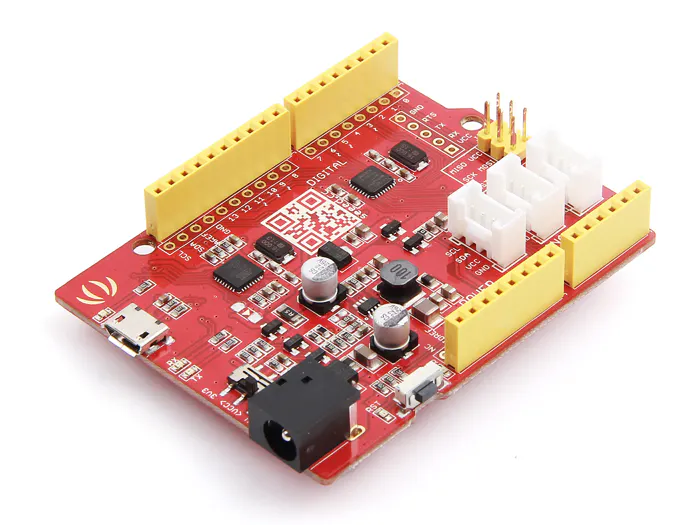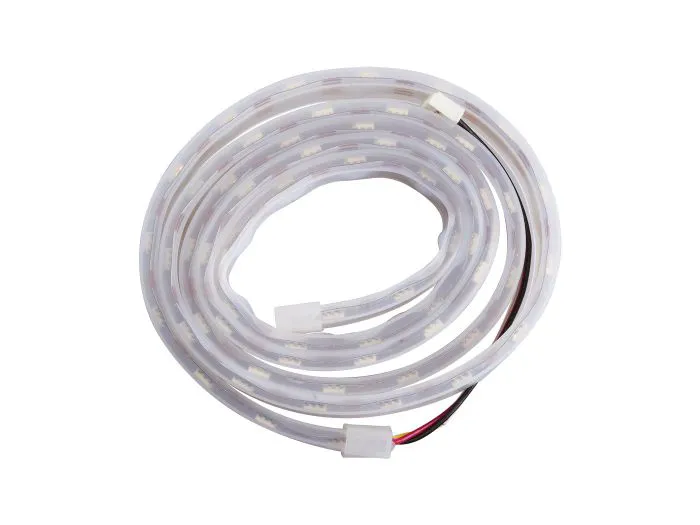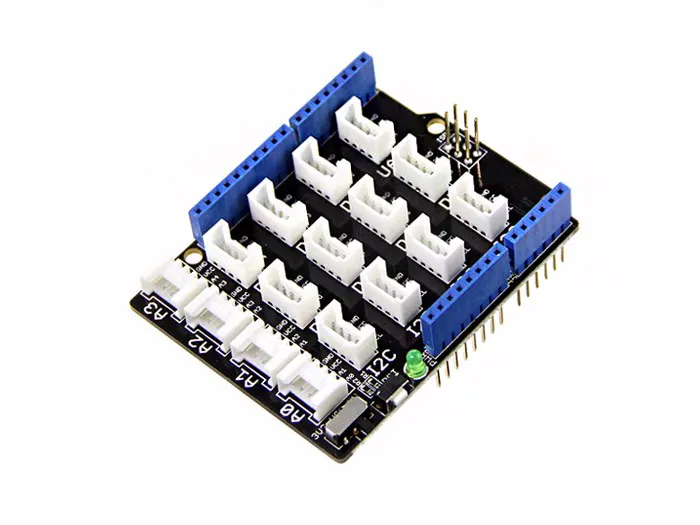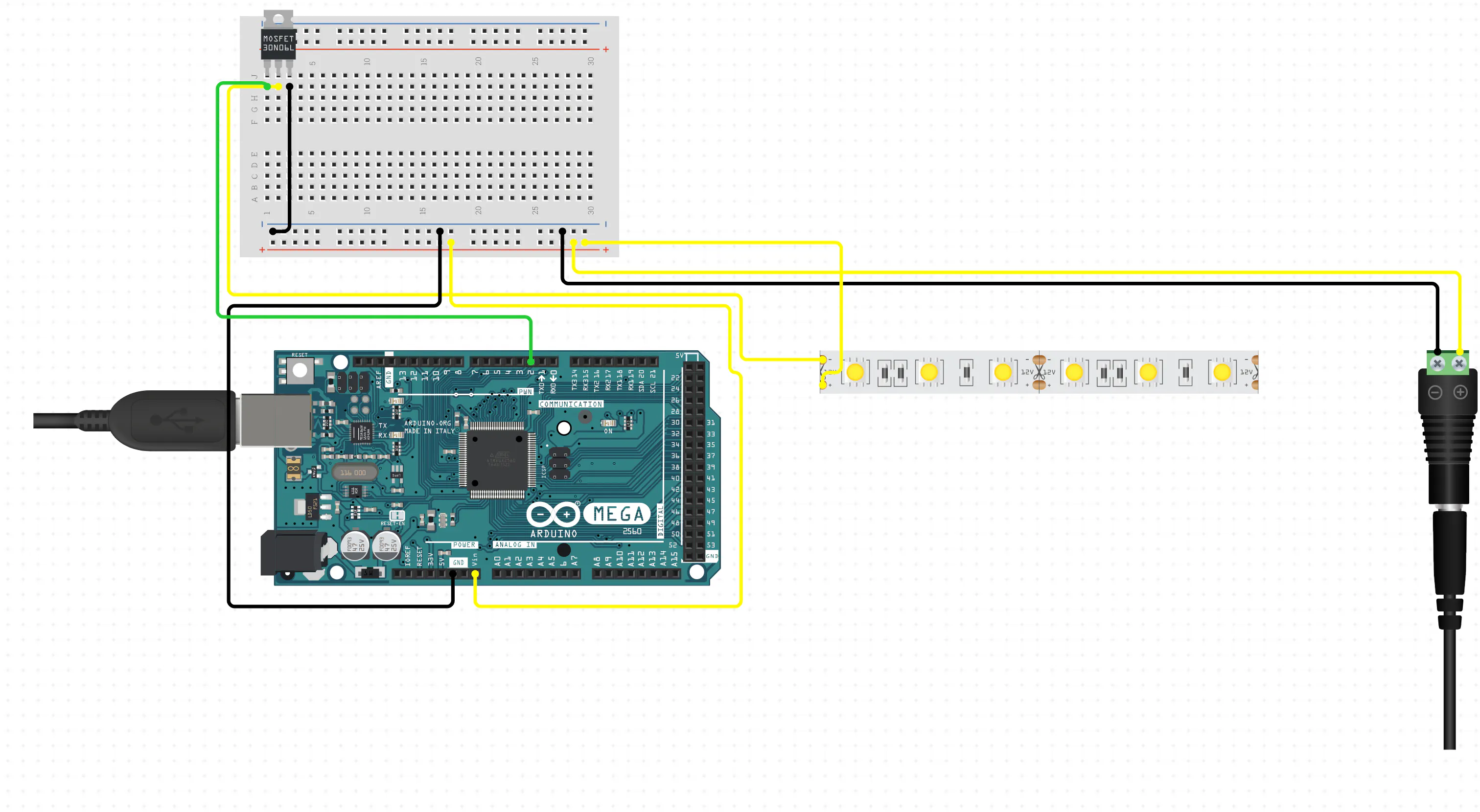# Visualize your bitcoin gain and loss with arduino & python

Get real-time feedback of your crypto currency investment without keeping a close eye on the trading data. Let python and arduino help you!

BeginnerFull instructions provided2 hours499

## Things used in this project

### Hardware componentsSeeeduino V4.2
×1Seeed Waterproof WS2813 RGB LED Strip Waterproof
×1Seeed Base Shield V2
×1

### Software apps and online servicesArduino IDEMicrosoft VS Code

## Schematics

### arduino and led strip circuit diagram## Code

### Arduino

Arduino
Get data from python and control the led strip
```#include <FastLED.h>

#define NUM_LEDS 30
#define DATA_PIN 6
CRGB leds[NUM_LEDS];
String dataStr;      // a variable to read incoming serial data into
int data;
int range;
void setup() //set up the strip
{
Serial.begin(9600);
FastLED.setBrightness(30);
FastLED.show();
}

void loop() {
if (Serial.available() > 0) {
FastLED.clear();
data = dataStr.toInt();
if (data == 30 ) buy();
if (data == -30) sell();
if (data <= 0) {
for (int i = 0 ; i <= abs(data) - 1 ; i++) // based on current loss, turn on certain amout of red leds
{
leds[i].setRGB(0, 255, 0);
FastLED.show();
delay(10);
}
}
if (data > 0) {
for (int i = 0 ; i <= abs(data) - 1 ; i++)
{  // based on current gain, turn on certain amout of green leds
leds[i].setRGB(255, 0, 0);
FastLED.show();
delay(10);
}
}
}
}

void sell() //sell signal
{
for (int i = NUM_LEDS - 1; i >= 0 ; i--)
{
leds[i].setRGB(0, 255, 0);
FastLED.show(); // This sends the updated piaXel color to the hardware.
delay(10);
}
}

for (int i = 0; i < NUM_LEDS; i++)
{

leds[i].setRGB(255, 0, 0);
FastLED.show(); // This sends the updated piaXel color to the hardware.
delay(10);
}
}

{
for (int i = 0; i < NUM_LEDS; i++)
{
leds[i].nscale8(250);
}
}
```

### Python

Python
Fetch real-time bitcoin data and calculate my return of investment
```import requests
import serial
import time

'user-agent': 'Mozilla/5.0 (Macintosh; Intel Mac OS X 10_15_2) AppleWebKit/537.36 (KHTML, like Gecko) Chrome/79.0.3945.117 Safari/537.36'}

arduino = serial.Serial('/dev/cu.usbmodem14401', 9600)
time.sleep(1)

url = 'https://www.okex.me/api/v1/ticker.do?symbol=btc_usdt'

#set up your initial investment here
basePrice = 8850.0
coins = 10
targetGain = 0.005
targetLoss = 0.005

def get_latest_crypto_price(): #get real-time bitcoin price
data = res.json()['ticker']
btcPrice = float(data.get('last'))
return btcPrice

def main():

last_price = -1
while True:

price = get_latest_crypto_price()
if price != last_price:
if price <= basePrice:
loss = (price - basePrice)/basePrice
netLoss = coins * (basePrice - price) #calculate loss
print('current bitcoin price is {}. Your loss is {} dollar'.format(
price, netLoss))
rangeLed = int(loss * 30 / targetLoss) #tell arduino how many leds to turn on

if rangeLed <= -30:
rangeLed = -30
if rangeLed == 0:
rangeLed = -1

arduino.write(str(rangeLed))
if price > basePrice:
gain = (price - basePrice)/basePrice
netGain = coins * (price - basePrice) #calculate gain
print('current bitcoin price is {}. Your gain is {} dollar'.format(
price, netGain))
rangeLed = int(gain * 30 / targetGain)
if rangeLed >= 30:
rangeLed=30
if rangeLed == 0:
rangeLed=1

arduino.write(str(rangeLed))
last_price=price
time.sleep(3)

main()
```

## Credits

### memoryleak

5 projects • 5 followers
A soft and hardware engineer motivated by interest and fun